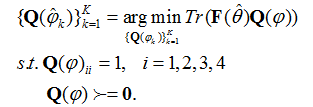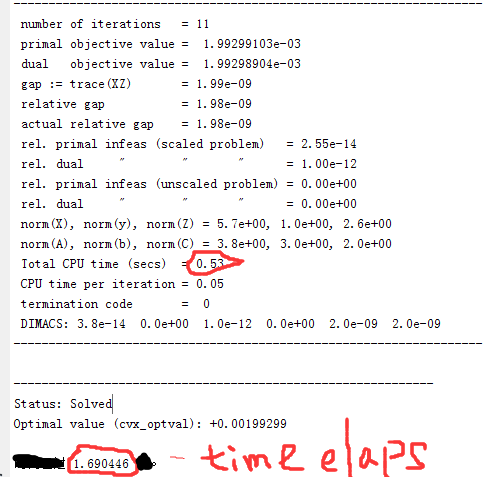# Why the complexity is so high

Dear Prof.
When I try to run the following SDP models in a Matlab m file:
cvx_begin
variable Q(4,4) complex semidefinite
minimize(abs(trace(F(:,:,k)*Q)));
subject to
Q(1,1) == 1;
Q(2,2) == 1;
Q(3,3) == 1;
Q(4,4) == 1;
cvx_end
but the CPU runtime is so expensive that nobody can tolerate it. Anyone can help me resolve this problem. Thanks a lot!!!

BTW: the math model is givenI suggest you post complete log output. Maybe also saying what K is a good idea.

K is fixed integer, and F(theta) is known ahead of time. and I want to know what is the worst time complexity for this problem, please help me to analyze theoretically, thanks so much!

I think @Erling was suggesting that if you tell us what values of K are in the problems you want to solve, and show solver output for specific examples, he or other forum readers can help assess and diagnose your runtimes.

K=3 in my case, or you can set K=1, my concern is other parts between cvx_begin and cvx_end appears to be time exausting assuming the above problem is posed and unchanged

Show us the output. Even better if you can also show us the input.

Roughly speaking, runtime can be divided into CVX processing time and solver time. We have what that division is in your case unless you provide more information.

Thanks Mark.
What is the percentage of CVX processing time since runtime contains two parts: efficient solver time and unefficient processing time?

% for k=1:3
% cvx_begin
% variable Q(4,4) complex semidefinite
% minimize(abs(trace(F(:,:,k)Q))); % F(:,:,k) is 4-by-4 matrix
% subject to
% diag(Q) == 1;
% cvx_end
% Theta_QCCQP_Est(k)=acosd(phase(Q(1,2))lambda/(2pi
d));
% end

Percentage of wall clock time going to CVX processing time vs. solver time varies depending on the problem and solver. How long does it take until solver output starts appearing?

I don’t think you’re going to get much help unless you answer the questions of people trying to help you.

Mark
The above is the output after I run the codes between cvx_begin and cvx_end at single step

Are you complaining about the Total CPU time = 1.21 sec? Is there a long wait before solver output begins?

It looks like the solver got the job done.

If you have access to Mosek, try that. It may be faster. Plus Mosek staff who read this forum can better understand the nuances of the solver output from their own code.

Yes, the total CPU time is too long, however, I have no idea on how to obtain specific waiting time that is I want to really know before solver output begins. Any tech tool or built-in function is available for that? That way, I can estimate the solver running time accurately, so that the theoretical analysis result coincides with the computer output running time

Hi, Mark,
After I run the following codes, note: tic and toc pair are added
tic;
cvx_begin
variable Q(4,4) complex semidefinite
minimize(abs(trace(F(:,:,k)*Q)));
subject to
diag(Q) == 1;
cvx_end
toc;
the resulting output is as followswhether the above result means that 0.57s is the solver running time, and 1.69s is the total time elaped, that is, the CVX processing time is 1.69-0.57=1.12s? is that ture?

Presuming the solver time is honest, I think yes, more or less.

CVS is never the fastest way in computer time to solve a problem. You can incur a time penalty for its convenience in saving human development time and error propensity.

Thanks Mark,
I think your judgement makes sense.
theoretically, the compelexity of CVX solver is O{N^3}. N is the number of variables only? I mean that constraints (equality or unequality constraints) should not be taken into account when estimte compelexity using O{N^3}

Cvx is not solver but an interface to an optimizer such as SDPT3, Mosek and SeDuMi…

Almost surely the Cvx by itself has strong polynomial complexity. You most likely refer to the complexity of the underlying optimizer which you have gotten wrong.